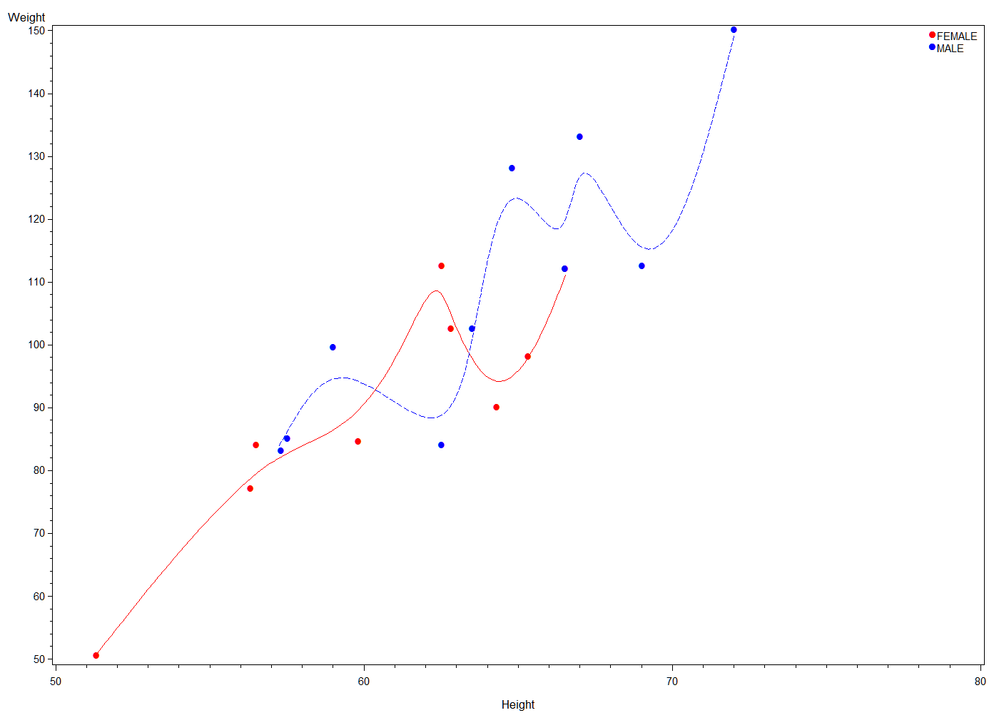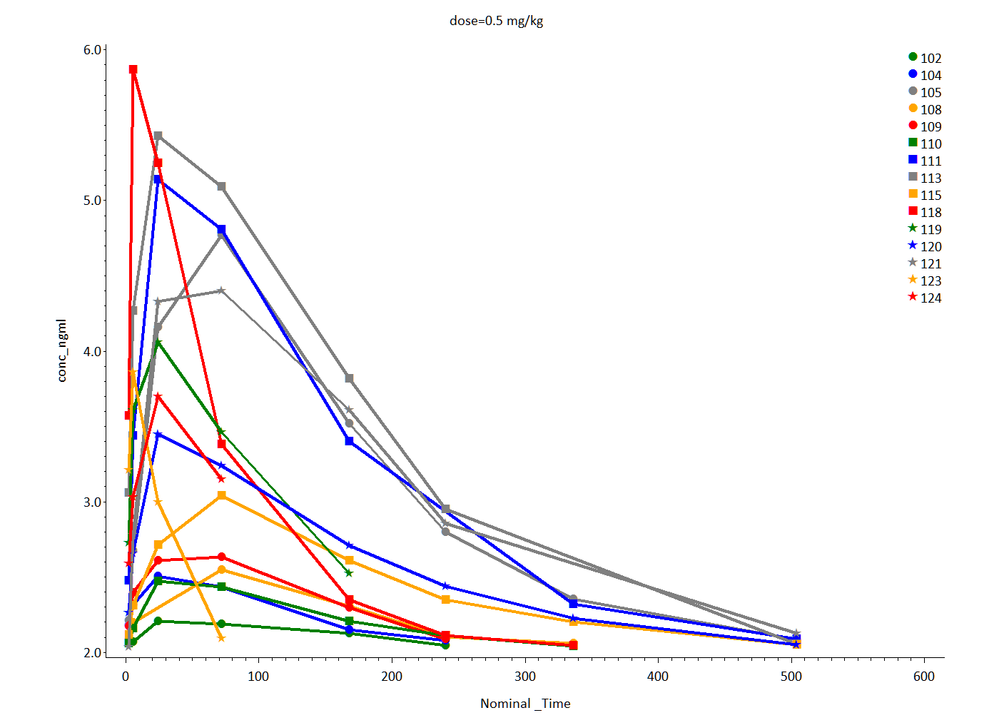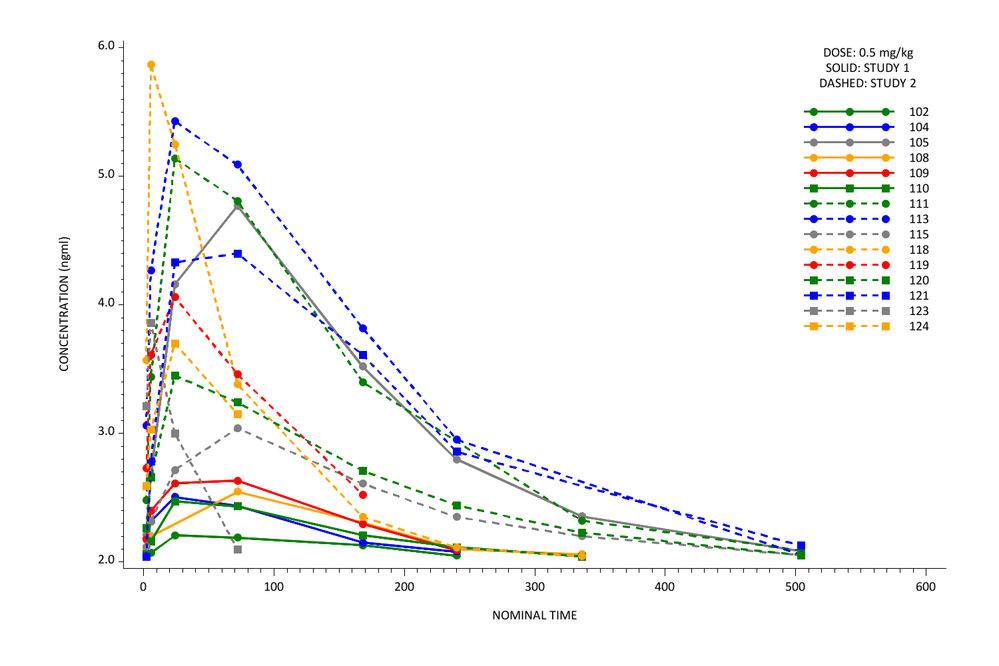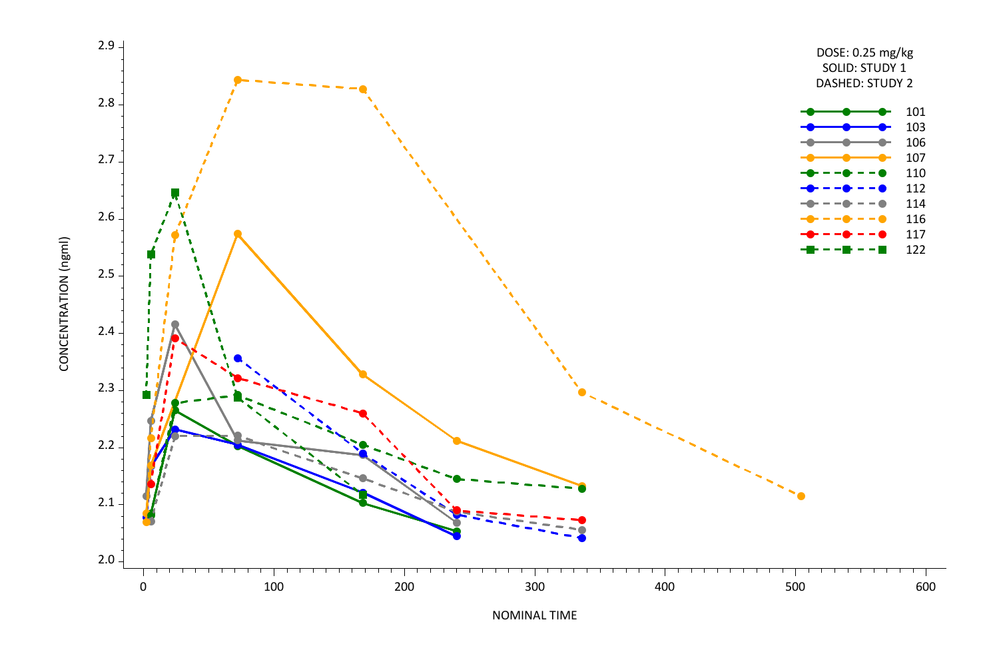## Proc Gplot line type option in symbol

I need to give different line option depending upon the variable study in my dataset i.e. if the dataset has study = "study1" then the line=1 and if the data has study="study2" then line=2. Also, I am plotting the graph for various doses. Is there a way I can specify in SAS to change the line option ?

8 REPLIES 8MikeZdeb
Rhodochrosite | Level 12

## Re: Proc Gplot line type option in symbol

hi ... here's an example where line color and type varies by the value of the variable sex (there are 46 line patterns, 1 is solid, etc.)

look at the SYMBOL statements ... l=1 is short for line=1

proc sort data=sashelp.class out=class;

by height;

run;

goptions reset=all;

legend1 position=(top inside right) label=none across=1 shape=symbol(.0005,1) value=('FEMALE' 'MALE');

symbol1 v='6c'x f='wingdings' c=red  i=smp66 l=1;

symbol2 v='6c'x f='wingdings' c=blue i=smp66 l=3;

proc gplot data=class;

plot weight*height=sex / legend=legend1;

run;

quit;

ps  lots of info in a SUGI paper (http://www2.sas.com/proceedings/sugi31/239-31.pdf)DanH_sas
SAS Super FREQ

## Re: Proc Gplot line type option in symbol

Hey,

Take a look at these two blog posts. I deal with this very issue and how you can make this happen using ODS graphics.

Hope this helps!

Dan

http://blogs.sas.com/content/graphicallyspeaking/2012/02/27/roses-are-red-violets-are-blue/

http://blogs.sas.com/content/graphicallyspeaking/2012/03/01/roses-are-red-violets-are-blue-part-2/MikeZdeb
Rhodochrosite | Level 12

## Re: Proc Gplot line type option in symbol

hi ... what do you mean by "line statement"

do you really mean SYMBOL statement ?

also, this ...

plot conc_ngml*Nominal_Time_day=subject_alias;

you are putting 10 lines on one plot, 15 on another =  unreadable yes/no?MikeZdeb
Rhodochrosite | Level 12

## Re: Proc Gplot line type option in symbol

hi ... using your data (too many lines to make any sense ???) ...

libname x 'z:\gplot data.xls';

proc sort data=x."'#LN00453\$'"n out=x;

by dose;

run;

goptions reset=all ftext='calibri' htext=2 gunit=pct;

symbol1 i=join v='6c'x f='wingdings' w=4 h=2 color=green;

symbol2 i=join v='6c'x f='wingdings' w=4 h=2 color=blue;

symbol3 i=join v='6c'x f='wingdings' w=4 h=2 color=grey;

symbol4 i=join v='6c'x f='wingdings' w=4 h=2 color=orange;

symbol5 i=join v='6c'x f='wingdings' w=4 h=2 color=red;

symbol6  i=join v='6e'x f='wingdings' w=4 h=2 color=green;

symbol7  i=join v='6e'x f='wingdings' w=4 h=2 color=blue;

symbol8  i=join v='6e'x f='wingdings' w=4 h=2 color=grey;

symbol9  i=join v='6e'x f='wingdings' w=4 h=2 color=orange;

symbol10 i=join v='6e'x f='wingdings' w=4 h=2 color=red;

symbol11 i=join v='ab'x f='wingdings' w=4 h=2 color=green;

symbol12 i=join v='ab'x f='wingdings' w=4 h=2 color=blue;

symbol13 i=join v='ab'x f='wingdings' w=4 h=2 color=grey;

symbol14 i=join v='ab'x f='wingdings' w=4 h=2 color=orange;

symbol15 i=join v='ab'x f='wingdings' w=4 h=2 color=red;

legend1 position=(top inside right) mode=protect across=1 label=none shape=symbol(.0005,2)pct;

axis1 offset=(2,2)pct;

axis2 label=(a=90);

title1 a=90 ls=2;

title2 a=-90 ls=2;

proc gplot data = x;

plot conc_ngml*Nominal__Time=subject__alias / legend=legend1 haxis=axis vaxis=axis2 noframe;

by dose;

format conc_ngml 4.1;

run;

quit;## Re: Proc Gplot line type option in symbol

Thanks for the code. Yes, I know too many lines on one plot will be very messy. But, I need to prepare this plot to see the difference between the two studies.

Is it also possible to get dotted lines (i.e using line=2) option whenever a subject is coming from study 2 and line = 1whenever a subject is coming from study 1?MikeZdeb
Rhodochrosite | Level 12

## Re: Proc Gplot line type option in symbol

hi ... the attached SAS code produced the two attached plots

I just took out the "by dose" and did two separate plots, changing the symbols from plot1 to plot2 to conform with the number of people in each study

I think it's correct, but you should check the allocation of people to study## Re: Proc Gplot line type option in symbol

Thanks a lot for your help.

So, we have to create two codes for different dose levels. I was hoping to make one code and tweak the symbol option so that it takes line=1 for study1 and line=2 for study2, maybe by using some macro etc. Because I will having more than 2 dose groups in my dataset and I wanted to avoid repeating my code.MikeZdeb
Rhodochrosite | Level 12

## Re: Proc Gplot line type option in symbol

hi ... given the choice of trying to write SAS code that would figure out the SYMBOL statement based on the data versus just doing with I did, I would say that the choice depends on how often you are going to do this

for the two plots, the effort of cutting/pasting code took a LOT less time that writing SAS code that would write SAS code for

or "when to hold 'em, when to fold 'em"

Discussion stats
• 8 replies
• 12883 views
• 7 likes
• 3 in conversation